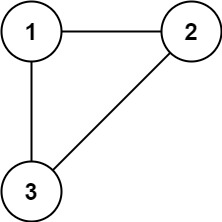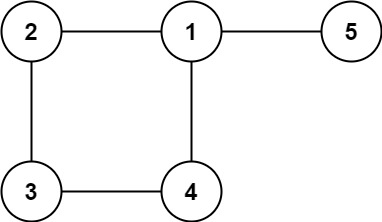# LeetCode Redundant Connection Solution

In this problem, a tree is an undirected graph that is connected and has no cycles.

You are given a graph that started as a tree with `n` nodes labeled from `1` to `n`, with one additional edge added. The added edge has two different vertices chosen from `1` to `n`, and was not an edge that already existed. The graph is represented as an array `edges` of length `n` where `edges[i] = [ai, bi]` indicates that there is an edge between nodes `ai` and `bi` in the graph.

Return an edge that can be removed so that the resulting graph is a tree of `n` nodes. If there are multiple answers, return the answer that occurs last in the input.

Example 1:Input: `edges = [[1,2],[1,3],[2,3]]`
Output: `[2,3]`

Example 2:Input: `edges = [[1,2],[2,3],[3,4],[1,4],[1,5]]`
Output: `[1,4]`

Constraints:

• `n == edges.length`
• `3 <= n <= 1000`
• `edges[i].length == 2`
• `1 <= ai < bi <= edges.length`
• `ai != bi`
• There are no repeated edges.
• The given graph is connected.

## Solution

We will apply Kruskal's algorithm using Union Find on this special graph where all the edges have equal weight (no sorting needed!). Instead of discarding an edge when we find a cycle, we return this edge as the answer. Moreover, the weight of the path is irrelevent to the problem, so we will not keep track. We follow the same implementation as finding the minimum spanning tree using Kruskal's algorithm. When we find an edge `e` such that its two ends are in the same component, we know that it will create a cycle, hence `e` is the extra edge added into the tree.

#### Implementation

``````1def findRedundantConnection(edges: List[List[int]]) -> List[int]:
2    id = {}
3    def find(x):
4        y = id.get(x, x)
5        if y != x:
6            id[x] = y = find(y)
7        return y
8    def union(x, y):
9        id[find(x)] = find(y)
10
11    for e in edges:
12        if find(e) == find(e):  # two ends in the same component
13            return e
14        union(e, e)             # join two components``````